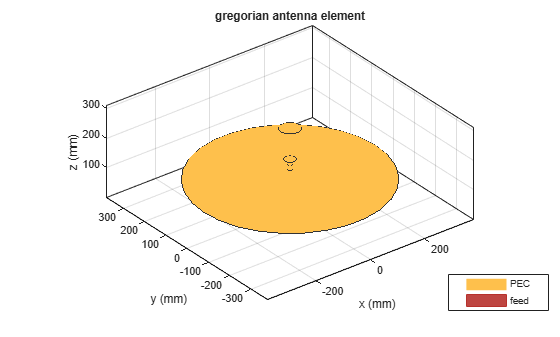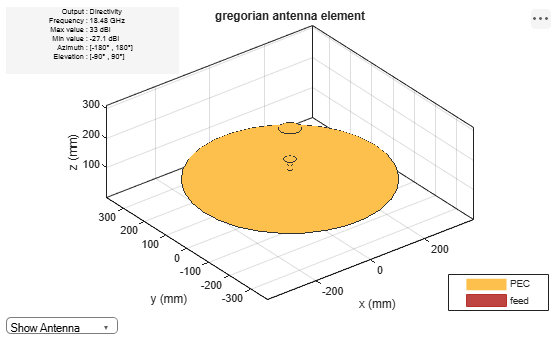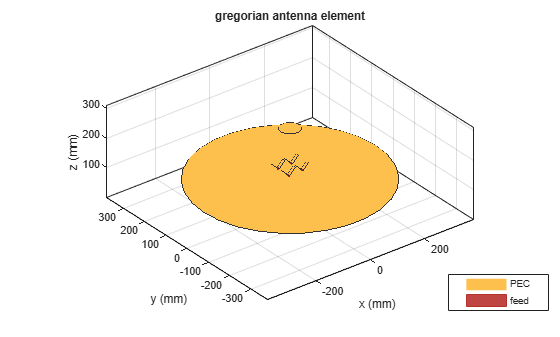# gregorian

Create Gregorian antenna

Since R2019b

## Description

The `gregorian` object creates a horn conical fed Gregorian antenna. A Gregorian antenna is a parabolic antenna. In this antenna, the feed antenna is mounted at or behind the surface of the main parabolic reflector and aimed at the sub-reflector. This antenna is used in radio telescopes and communication satellites. For more information see, Architecture of Gregorian Antenna.## Creation

### Syntax

``ant = gregorian``
``ant = gregorian(Name=Value)``

### Description

example

````ant = gregorian` creates a horn conical fed Gregorian antenna with a default operating frequency of 18.48 GHz. This antenna gives maximum gain when operated at 18.3 GHz.```
````ant = gregorian(Name=Value)` creates a Gregorian antenna, with additional Properties specified by one or more name–value arguments. `Name` is the property name and `Value` is the corresponding value. You can specify several name-value arguments in any order as `Name1`= `Value1`, `...`, `NameN`=`ValueN`. Properties not specified retain their default values.For example, `ant = gregorian(FocalLength=[0.4 0.22])` creates a Gregorian antenna with the main reflector of focal length 0.4 m and the sub-reflector of focal length 0.22 m.```

## Properties

expand all

Exciter antenna or array type, specified as an antenna object, an array object, measured pattern data of an antenna, or an empty array. Except for reflector and cavity antenna elements, you can use any Antenna Toolbox™ antenna or array element as an exciter. To create the reflector backing structure without the exciter, specify this property as an empty array.

Example: `dipole`

Example: `linearArray(Element=patchMicrostrip)`

Example: `measuredAntenna`

Example: `[]`

Radius of the main and sub-reflector, specified as a two-element vector with each element unit in meters. The first element specifies the radius of the main reflector, and the second element specifies the radius of the sub-reflector.

Example: `Radius=[0.4 0.2]`

Data Types: `double`

Focal length of the main and sub-reflector, specified as a two-element vector with each element unit in meters. The first element specifies the focal length of the main reflector, and the second element specifies the focal length of the subreflector.

Example: `FocalLength=[0.35 0.2]`

Data Types: `double`

Lumped elements added to the antenna feed, specified as a lumped element object. You can add a load anywhere on the surface of the antenna. By default, the load is at the feed. For more information, see `lumpedElement`.

Example: `Load=lumpedelement`. `lumpedelement` is the object for the load created using `lumpedElement`.

Example: `lumpedElement(Impedance=75)`

Tilt angle of the antenna in degrees, specified as a scalar or vector. For more information, see Rotate Antennas and Arrays.

Example: `90`

Example: `Tilt=[90 90]`,`TiltAxis=[0 1 0;0 1 1]` tilts the antenna at 90 degrees about the two axes defined by the vectors.

Data Types: `double`

Tilt axis of the antenna, specified as one of these values:

• Three-element vector of Cartesian coordinates in meters. In this case, each coordinate in the vector starts at the origin and lies along the specified points on the x-, y-, and z-axes.

• Two points in space, specified as a 2-by-3 matrix corresponding to two three-element vectors of Cartesian coordinates. In this case, the antenna rotates around the line joining the two points.

• `"x"`, `"y"`, or `"z"` to describe a rotation about the x-, y-, or z-axis, respectively.

Example: `[0 1 0]`

Example: `[0 0 0;0 1 0]`

Example: `"Z"`

Data Types: `double` | `string`

Solver for antenna analysis, specified as a string. Default solver is `"MoM-PO"`(Method of Moments-Physical Optics hybrid). Other supported solvers are: `"MoM"` (Method of Moments), `"PO"` (Physical optics) or `"FMM"` (Fast Multipole Method).

Example: `SolverType`=`"MoM"`

Data Types: `string`

## Object Functions

 `show` Display antenna, array structures, shapes, or platform `solver` Access FMM solver for electromagnetic analysis `axialRatio` Axial ratio of antenna `beamwidth` Beamwidth of antenna `charge` Charge distribution on antenna or array surface `current` Current distribution on antenna or array surface `design` Design prototype antenna or arrays for resonance around specified frequency or create AI-based antenna from antenna catalog objects `EHfields` Electric and magnetic fields of antennas or embedded electric and magnetic fields of antenna element in arrays `impedance` Input impedance of antenna or scan impedance of array `mesh` Mesh properties of metal, dielectric antenna, or array structure `meshconfig` Change meshing mode of antenna, array, custom antenna, custom array, or custom geometry `optimize` Optimize antenna or array using SADEA optimizer `pattern` Plot radiation pattern and phase of antenna or array or embedded pattern of antenna element in array `patternAzimuth` Azimuth plane radiation pattern of antenna or array `patternElevation` Elevation plane radiation pattern of antenna or array `rcs` Calculate and plot radar cross section (RCS) of platform, antenna, or array `returnLoss` Return loss of antenna or scan return loss of array `sparameters` Calculate S-parameters for antennas and antenna arrays `vswr` Voltage standing wave ratio (VSWR) of antenna or array element

## Examples

collapse all

Create and view a default Gregorian antenna.

`ant = gregorian`
```ant = gregorian with properties: Exciter: [1x1 hornConical] Radius: [0.3175 0.0330] FocalLength: [0.2536 0.1416] Tilt: 0 TiltAxis: [1 0 0] Load: [1x1 lumpedElement] SolverType: 'MoM-PO' ```
`show(ant)`Plot the radiation pattern of the antenna at 18.48 GHz.

`pattern(ant,18.48e9)`Create an array of vee dipole antennas.

```e = dipoleVee(ArmLength=[0.03 0.03],Width=0.01); arr = rectangularArray(Element=e,RowSpacing=0.05,ColumnSpacing=0.05);```

Create a Gregorian antenna with rectangular array as exciter

```ant = gregorian(Exciter=arr); show(ant)```